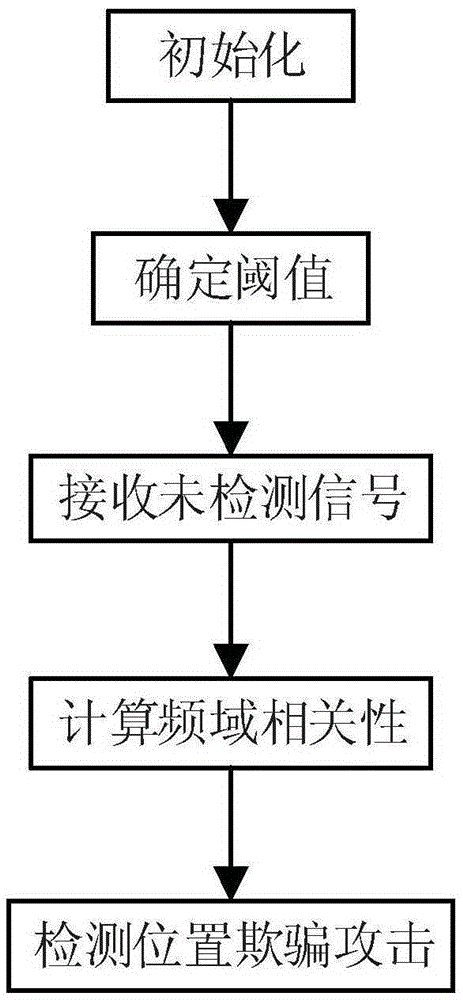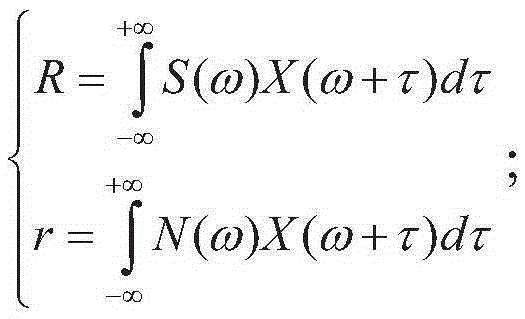# Position spoofing attack detection method for frequency modulation signal indoor positioning system

## An FM signal, indoor positioning technology, applied in the field of communication, can solve the problem of position information acquisition error, positioning system position determination error and other problems

Active Publication Date: 2016-03-16
XIDIAN UNIV
4 Cites 0 Cited by

## AI-Extracted Technical Summary

### Problems solved by technology

 A location spoofing attack refers to an attacker tampering with the location information of a normal user's location, so that the normal user obtains the location information tampered with by the attacker when the normal user needs to obtain the location information, resulting in an error in obtaining the location information
The position spoofing attack of the FM signal indoor positioning system is based on the signal strength of the target position and th...
View more

## Abstract

The invention discloses a position spoofing attack detection method for a frequency modulation signal indoor positioning system, mainly to solve the problem that a position spoofing attack cannot be detected in a frequency modulation signal-based indoor positioning system. The method comprises steps of initialization, threshold determination, undetected signal receiving, frequency domain correlation calculation and position spoofing attack detection. Frequency domain characteristics of normal frequency modulation signals, carrier signals and natural noise signals are fully combined, time domain signals are converted into the frequency domain signals for processing, and the characteristic that the time domain signals in the position spoofing attack have irregular amplitude variation can be avoided; spectral cross-correlation is adopted for representing signal features, and high-efficiency judgment on attacked signals can be ensured; and an upper threshold and a lower threshold are used for dual judgment, and detection accuracy is improved.

Application Domain

Security arrangement

Technology Topic

Carrier signalIndoor positioning system +8

## Image

•••## Examples

• Experimental program(1)

### Example Embodiment

 The present invention will be further described below in conjunction with the accompanying drawings. It should be noted that this embodiment is based on the technical solution and provides detailed implementation and specific operation procedures, but the scope of protection of the present invention is not limited to this Examples.
 The application scenario of the present invention is an FM signal indoor positioning system, which includes FM signal base stations, attackers and normal users. The user calculates the cross-correlation between the received undetected signal and the frequency spectrum of the FM carrier signal, and uses the upper and lower thresholds to determine the signal type to obtain the detection result of the location spoofing attack.
 Such as figure 1 As shown, the specific steps of the present invention are as follows:
 Step 1. Initialization.
 1.1) The user uses its own antenna to receive 100 natural noise signals at the center of gravity frequency of the FM signal when the FM signal base station used for positioning is not working; when the FM signal base station used for positioning works, it receives 100 normal FM signals . It should be noted that 100 times is the better number of receptions. The more the number of receptions, the more accurate the value obtained, but the processing time will increase.
 1.2) Construct the carrier signal of the FM signal for positioning according to the following formula:
 x=Acos(2πft);
 Where x represents the carrier signal, A represents the amplitude, cos represents the cosine function, π represents the circumference of the circle, f represents the frequency, and t represents the time.
 Step 2. Determine the threshold.
 2.1) Use Fourier transform formula to calculate the frequency spectrum of natural noise signal, normal frequency modulation signal and carrier signal:
 X ( ω ) = ∫ - ∞ + ∞ x ( t ) e - j ω t d t S ( ω ) = ∫ - ∞ + ∞ s ( t ) e - j ω t d t N ( ω ) = ∫ - ∞ + ∞ n ( t ) e - j ω t d t ;
 Where X represents the carrier signal in the frequency domain, S represents the normal FM signal in the frequency domain, N represents the natural noise signal in the frequency domain, ω represents the angular frequency, ∫dt represents the integral over time t, and +∞ represents positive infinity, -∞ means negative infinity, carrier signal in x time domain, normal FM signal in s time domain, natural noise signal in n time domain, t means time, and e means natural base.
 2.2) Calculate the correlation coefficient between the frequency spectrum of the carrier signal and the normal FM signal received each time, and the correlation coefficient between the frequency spectrum of the carrier signal and the natural noise signal received each time:
 R = ∫ - ∞ + ∞ S ( ω ) X ( ω + τ ) d τ r = ∫ - ∞ + ∞ N ( ω ) X ( ω + τ ) d τ ;
 Where R represents the correlation coefficient between the frequency spectrum of the carrier signal and the normal FM signal, ∫dτ represents the integral of the parameter τ, +∞ represents positive infinity, -∞ represents negative infinity, S represents the normal FM signal in the frequency domain, and ω represents the angular frequency , X represents the carrier signal in the frequency domain, r represents the correlation coefficient between the frequency spectrum of the carrier signal and the natural noise signal, and N represents the natural noise signal in the frequency domain.
 2.3) Set the average value of the frequency spectrum correlation coefficient of the carrier signal and all received normal FM signals as the upper threshold, and set the average value of the frequency spectrum correlation coefficient of the carrier signal and all received natural noise signals as the lower threshold value.
 Step 3. Receive undetected signals.
 The user uses his own antenna to receive undetected signals on the frequency of the FM signal used for positioning. The undetected signal contains three possibilities: normal FM signal, natural noise signal and signal after being attacked by location spoofing.
 Step 4. Calculate the frequency domain correlation.
 4.1) Use the Fourier transform formula to calculate the frequency spectrum of the undetected signal:
 G ( ω ) = ∫ - ∞ + ∞ g ( t ) e - j ω t d t
 Where G represents the undetected signal in the frequency domain, ω represents the angular frequency, ∫dt represents the integral over time t, +∞ represents positive infinity, -∞ represents negative infinity, g represents the carrier signal in the time domain, t represents time, e represents the natural base, j represents the imaginary unit.
 4.2) Calculate the correlation coefficient between the frequency spectrum of the undetected signal and the carrier signal:
 W = ∫ - ∞ + ∞ G ( ω ) X ( ω + τ ) d τ ;
 Where W represents the spectral correlation coefficient between the undetected signal and the carrier signal, ∫dτ represents the integral of the parameter τ, +∞ represents positive infinity, -∞ represents negative infinity, G represents the undetected signal in the frequency domain, and ω represents the angular frequency , X represents the carrier signal in the frequency domain.
 Step 5. Detect location spoofing attacks.
 Determine whether the correlation coefficient between the frequency spectrum of the carrier signal and the undetected signal is less than the upper threshold. If yes, determine whether the correlation coefficient between the frequency spectrum of the carrier signal and the undetected signal is greater than the lower threshold. If yes, it means that the undetected signal is a signal attacked by location spoofing. A location spoofing attack occurs in the FM signal indoor positioning system; on the contrary, it means that the undetected signal is a natural noise signal; on the contrary, it means that the undetected signal is a normal FM signal. The test is over.
 For those skilled in the art, various corresponding changes and modifications can be made based on the above technical solutions and concepts, and all these changes and modifications should be included in the protection scope of the claims of the present invention.

## PUM## Description & Claims & Application Information

We can also present the details of the Description, Claims and Application information to help users get a comprehensive understanding of the technical details of the patent, such as background art, summary of invention, brief description of drawings, description of embodiments, and other original content. On the other hand, users can also determine the specific scope of protection of the technology through the list of claims; as well as understand the changes in the life cycle of the technology with the presentation of the patent timeline. Login to view more.
Who we serve
• R&D Engineer
• R&D Manager
• IP Professional
Why Eureka